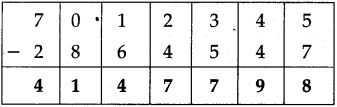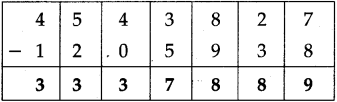# Maharashtra Board Class 5 Maths Solutions Chapter 3 Addition and Subtraction Problem Set 11

Balbharti Maharashtra Board Class 5 Maths Solutions Chapter 3 Addition and Subtraction Problem Set 11 Textbook Exercise Important Questions and Answers.

## Maharashtra State Board Class 5 Maths Solutions Chapter 3 Addition and Subtraction Problem Set 11

Question 1.
Subtract the following:

(1) 8,57,513 – 4,82,256
Solution: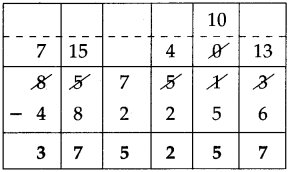3,75,257(2) 13,17,519 – 10,07,423
Solution: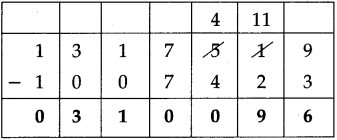3,10,096

(3) 68,34,501 – 23,57,823
Solution: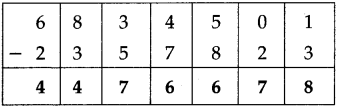44,76,678

(4) 45,43,827 – 12,05,938
Solution: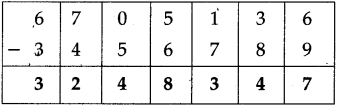33,37,889(5) 70,12,345 – 28,64,547
Solution: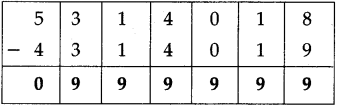41,47,798

(6) 38,01,213 – 37,54,648
Solution: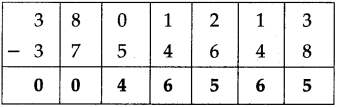46,565

Study the following word problem.

In 2001, the population of a city was 21,43,567. In 2011, it was 28,09,878. By how much did the population grow?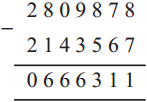The population grew by 6,66,311.Subtract the following:

(1) 53,14,018 – 43,14,019
Solution: